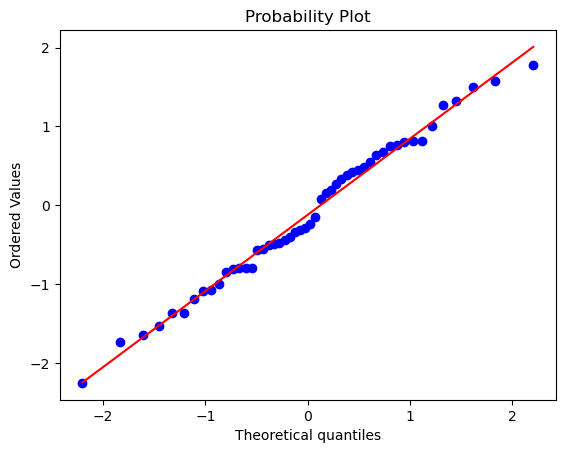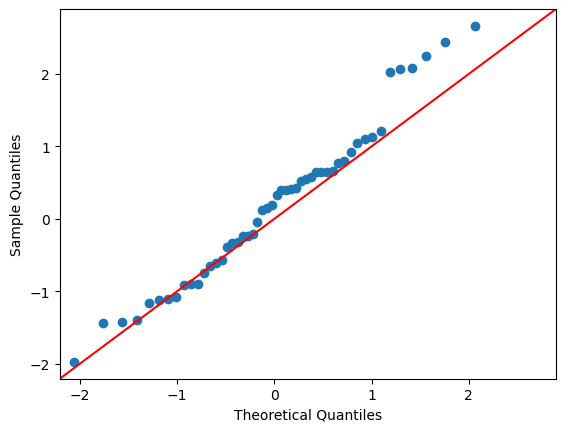# How to create a QQ-plot

## Description

We often want to know whether a set of data is normally distributed, so that we can deduce what inference tests are appropriate to conduct. If we have a set of data and want to figure out if it comes from a population that follows a normal distribution, one tool that can help is a QQ plot. How do we make and interpret one?

## Using SciPy, in Python

View this solution alone.

We’re going to use some fake data here by generating random numbers, but you can replace our fake data with your real data in the code below.

1
2
3
# Replace this with your data, such as a variable or column in a DataFrame
import numpy as np
values = np.random.normal(0, 1, 50)  # 50 random values


If the data is normally distributed, then we expect that the QQ plot will show the observed values (blue dots) falling very clsoe to the red line (the quantiles for the normal distribution).

1
2
3
4
5
from scipy import stats
import matplotlib.pyplot as plt

stats.probplot(values, dist="norm", plot=plt)
plt.show()Our observed values fall pretty close to the reference line. In this case, we expected that, because we created fake data that was normally distributed. But for real data, it may not stay so close to the red line.

See a problem? Tell us or edit the source.

## Using statsmodels, in Python

View this solution alone.

We’re going to use some fake data here by generating random numbers, but you can replace our fake data with your real data in the code below.

1
2
3
# Replace this with your data, such as a variable or column in a DataFrame
import numpy as np
values = np.random.normal(0, 1, 50)  # 50 random values


If the data is normally distributed, then we expect that the QQ plot will show the observed values (blue dots) falling very clsoe to the red line (the quantiles for the normal distribution).

1
2
3
4
5
import statsmodels.api as sm
import matplotlib.pyplot as plt

sm.qqplot(values, line = '45')
plt.show()Our observed values fall pretty close to the reference line. In this case, we expected that, because we created fake data that was normally distributed. But for real data, it may not stay so close to the red line.

See a problem? Tell us or edit the source.

## Solution, in R

View this solution alone.

We’re going to use some fake data here by generating random numbers, but you can replace our fake data with your real data in the code below.

1
2
# Replace this with your data, such as a variable or column in a DataFrame
values <- c(4, 90, 85, 49, 34, 23, 17, 10, 20, 59, 100, 112, 46, 10, 4, 39, 24, 77, 63, 23, 67, 109, 70)


If the data is normally distributed, then we expect that the QQ plot will show the observed values (black circles) falling very clsoe to the red line (the quantiles for the normal distribution).

1
2
3
4
# Make a QQ plot for the data
qqnorm(values, pch = 1)
# Add the reference line representing what the data should look like if normally distributed
qqline(values, col = "red", lwd = 2)


Our observed values fall pretty close to the reference line. In this case, we expected that, because we created fake data that was normally distributed. But for real data, it may not stay so close to the red line.